# Binomial coefficients

The coefficients at the powers ofin the decomposition of the Newton binomial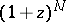. The binomial coefficients are denoted by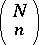or by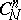, and are given by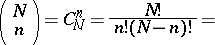(1)The first-mentioned notation is due to L. Euler; the notationappeared in the 19th century, and is probably connected with the interpretation of the binomial coefficientsas the number of distinguishable non-ordered combinations (cf. Combination) from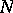different objects withobjects in each combination. Binomial coefficients are most conveniently written as numbers in the arithmetical triangle, or Pascal triangle, the construction of which is based on the following property of binomial coefficients: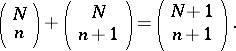(2)

Binomial coefficients, as well as the arithmetical triangle, were known concepts to the mathematicians of antiquity, in more or less developed forms. B. Pascal (l665) conducted a detailed study of binomial coefficients. The binomial coefficients are also connected by many useful relationships other than (2), for example:(3a)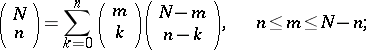(3b)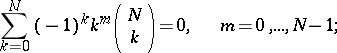(3c)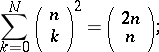(3d)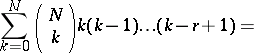(3e)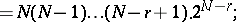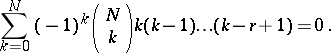(3f)

In particular, (3a)–(3f) yields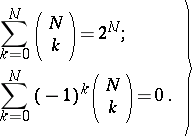(4)

The use of the Stirling formula yields approximate expressions for binomial coefficients. Thus, ifis much larger than: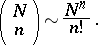In the case of a complex number, binomial coefficients are generalized according to the formula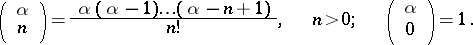In this generalization, some of the relations (2)–(4) are preserved, but usually in a modified form. For instance,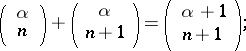For tables of binomial coefficients see , .